Next: Numerical simulation Up: Examples Previous: Repeated Measures

## Paired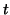-tests

Let us assume that for each of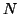subject there exist two measurements obtained under different conditions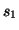,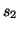and that we are interested in the significance of the mean group difference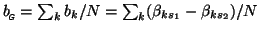. We will assume that the between-subject variance and the between-session variance is equal across subjects and conditions. Note that this is a notational simplification within this framework which might or might not become a necessary condition once we try to estimate the associated group-level parameters. Similar to the previous sections, we model this as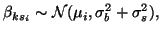and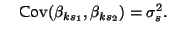Let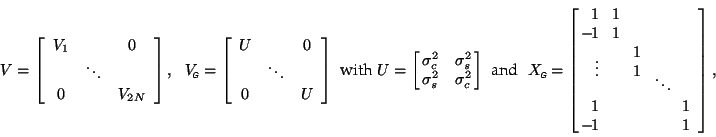where, again,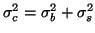and where the group design matrix,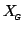, de-means the first level estimates for each subject. Assume for simplicity that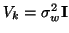,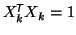and define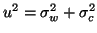. Then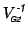will be block diagonal with blocks of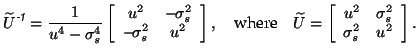Furthermore, let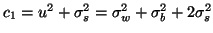and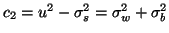. Then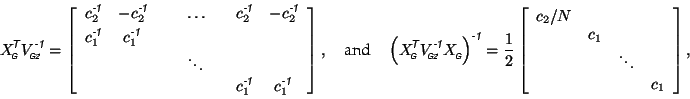so that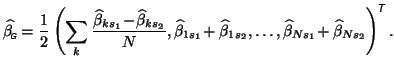Using, the group parameter estimate writes as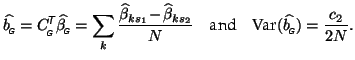As expected, the variance of the group level estimate no longer depends on the between-subject variance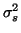. Note that this approach is equivalent to using a three level approach with an unpaired-test of de-meaned repeated measures.

SubsectionsNext: Numerical simulation Up: Examples Previous: Repeated Measures
Christian Beckmann 2003-07-16